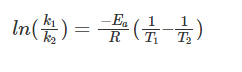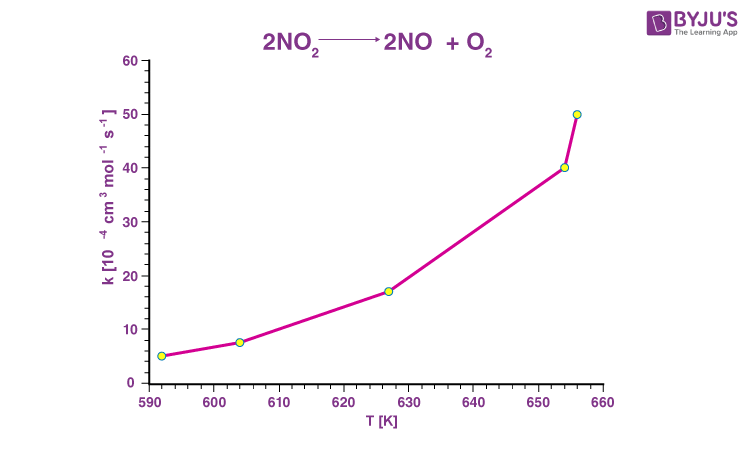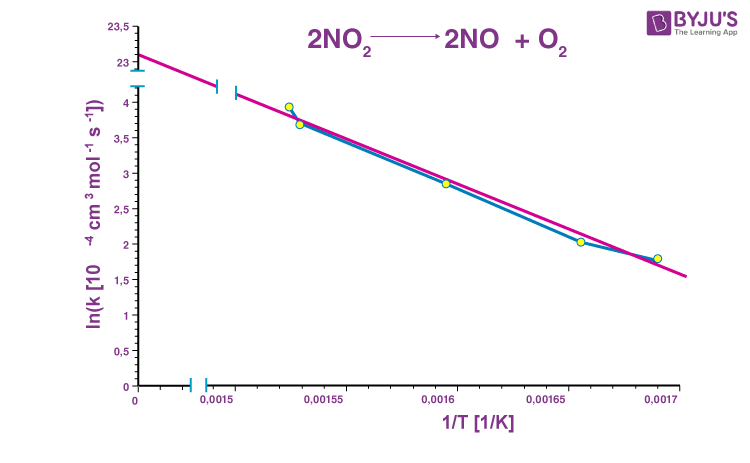Checkout JEE MAINS 2022 Question Paper Analysis : Checkout JEE MAINS 2022 Question Paper Analysis :

# Arrhenius Equation Questions

Chemical reactions occur more quickly at higher temperatures, as is well known. Milk spoils faster at room temperature than in the refrigerator; butter spoils faster in the summer than in the winter; and eggs hard boil faster at sea level than in the highlands.

At the molecular level, thermal energy relates motion to direction. Molecules move faster and collide more strongly as the temperature rises, significantly increasing the possibility of bond cleavages and rearrangements. Chemical reactions are often predicted to occur faster at higher temperatures and slower at lower temperatures, whether through collision theory, transition state theory, or basic common sense.

 Definition: The Arrhenius equation is an expression that shows how the rate constant (of a chemical reaction), absolute temperature, and the A factor are related (also known as the pre-exponential factor; can be visualised as the frequency of correctly oriented collisions between reactant particles).

## Arrhenius Equation Chemistry Questions with Solutions

Q1: Temperature dependence of reaction rates can be studied by plotting a graph between ___________

a) Concentration of reactants and temperature

b) Concentration of products and temperature

c) Rate constant and temperature

d) Rate of catalysis and temperature

Answer: c) Rate constant and temperature

Explanation: Plotting a graph between rate constant and temperature for various reactions can demonstrate the temperature dependence of reaction rates.

Q2: According to Arrhenius equation, rate constant(k) is proportional to ___________

a) Activation Energy (E)

b) eE

c) e1/E

d) e−E

Explanation: According to the Arrhenius equation, k = Ae−Ea/RT. So, rate constant(k) is proportional to e−E.

Q3: Which of the following methods is satisfactory only for a simple homogeneous reaction?

a) Half life period method

b) Integration method

c) Graphical method

d) Ostwald’s isolation method

Explanation: Integration method is suitable only for simple homogeneous reactions and results in wrong conclusions for complex reactions.

Q4: Which of the following methods is used to determine the order of the reaction in which two or more reactants take part?

a) Integration method

b) Half life period method

c) Ostwald’s isolation method

d) Graphical method

Explanation: Ostwald’s isolation technique is used to estimate the order of the reaction in which two or more reactants participate. The reaction order for interfacial charge recombination was determined due to the discovery of kinetic isolation conditions.

Q5: How does the half life period of a first order reaction vary with temperature?

a) It increases

b) It decreases

c) It remains the same

d) Both increases as well as decrease

Explanation: The rate constant is directly proportional to the half-life period of a first order reaction. As a result, as the temperature rises, it rises.

Q6: A reaction is of second order with respect to a reactant. How will the rate of reaction be affected if the concentration of this reactant is

(i) doubled, (ii) reduced to half?

(i) If [A] = 2a then Rate = K (2a)2 = 4 Ka2

∴ Rate of reaction becomes 4 times

(ii) If [A] = a/2 then Rate = K (a/2)2 = Ka2/4

∴ Rate of reaction will be 1/4th.

Q7: The activation energy of a chemical reaction is 100 kJ/mol and its A factor is 10 M−1s−1. Find the rate constant of this equation at a temperature of 300 K.

Given,

Ea = 100 kJ.mol−1 = 100000 J.mol−1

A = 10 M−1s−1, ln(A) = 2.3 (approx.)

T = 300 K

The rate constant can be calculated using the Arrhenius equation’s logarithmic form, which is:

ln k = ln(A) – (Ea/RT)

ln k = 2.3 – (100000 J.mol−1)/(8.314 J.mol−1.K−1)*(300K)

ln k = 2.3 – 40.1

ln k = −37.8

k = 3.8341*10−17 M−1s−1 (It is clear from the units of the ‘A’ factor that the reaction is a second-order reaction, with M−1s−1 as the unit of k)

Therefore, the value of the rate constant for the reaction at a temperature of 300K is approximately 3.8341*10−17 M−1s−1.

Q8: Write Arrhenius equation. Derive an expression for temperature variations.

Arrhenius equation is K = A e−Ea/RT

K = Rate constant, A = Pre-exponential factor

Ea= Activities energy, T = Temp. (Kelvin)

R = Gas constant.

The logarithmic form of the Arrhenius equation for a chemical reaction at two different temperatures T1 and T2, with corresponding rate constants K1 and K2, is:

ln K1 = ln(A) – Ea/RT1

ln K2 = ln(A) – Ea/RT2

The second equation can be rearranged to get the value of ln(A):

ln(A) = ln(K2) + Ea/RT2

Substituting the value of ln(A) in the equation for ln(K1), the following equations can be obtained:

ln(K1) = ln(K2) + Ea/RT2 – Ea/RT1

Shifting ln(K2) to the LHS, the value of ln(K1) – ln(K2) becomes:

ln(K1) – ln(K2) = Ea/RT2 – Ea/RT1

The LHS of the equation is of the form ln(x) – ln(y), which can be simplified to ln(x/y). In addition, the term ‘Ea/R‘ is a common factor in both RHS terms. As a result, the complete equation can be reduced to the following:Q9: Give the significance of Arrhenius Equation.

• This equation allows for the accounting of elements that influence the rate of reaction but cannot be determined using the rate law.
• The equation aids in determining the impact of the energy barrier, frequency, temperature, collision orientation, and catalyst presence.

Q10: At a temperature of 600 K, the rate constant of a chemical reaction is 2.75*10−8 M−1s−1. When the temperature is increased to 800K, the rate constant for the same reaction is 1.95*10−7M−1s−1. What is the activation energy of this reaction?

Given,

T1 = 600K

k1 = 2.75*10−8 M−1s−1.

T2 = 800K

K2 = 1.95*10−7M−1s−1

The following equation is obtained when the A factor is eliminated from the Arrhenius equation:

ln(k1/k2) = (−Ea/R)(1/T1 – 1/T2)

Substituting the given values in the equation, the value of Ea can be determined:

ln(2.75*10−8/1.95*10−7) = (−Ea/8.314 J.K−1.mol−1)*(0.00041K−1)

ln(0.141) = (Ea)*(−0.0000493) J−1.mol

Ea = (−1.958)/(−0.0000493)J.mol−1 = 39716 J.mol−1

The activation energy of the reaction is approximately 39716 J.mol−1.

Q11: Define the following:

(i) Elementary step in a reaction

(ii) Rate of a reaction

(i) Elementary step in a reaction: The reactions that occur in a single step are referred to as elementary reactions.

Example : Reaction between H2, and I2 to form 2HI

H2 + I2 → 2HI

(ii) Rate of a reaction: The rate of reaction is defined as the change in concentration of any of the reactants or products per unit time.

Q12: Mention the factors that affect the rate of a chemical reaction.

The factors that affect the rate of a chemical reaction are:

• Concentration of reactants
• Temperature
• Presence of catalysts
• Nature of reactants and products

Q13: A reaction is first order in A and second order in B :

(i) Write a differential rate equation.

(ii) How is the rate affected when the concentration of B is tripled?

(iii) How is the rate affected when the concentration of both A and B is doubled?

(i) Differential rate equation for the reaction is: rate (r) = k [A]1 [B]2

(ii) Differential rate equation for the reaction is: rate (r’) = k [A]1 [3B]2

r’/r = (k [A]1 [3B]2)/(k [A]1 [B]2) = 9 or r’ = 9r

Thus, the reaction rate will increase to 9 times.

(iii) Differential rate equation for the reaction is:

rate (r”) = k [2A]1 2[B]2 ; r”/r = (k [2A]1 2[B]2)/(k [A]1 [B]2)= 8 or r” = 8r

Thus, the reaction rate will increase to 8 times.

Q14: In Arrhenius equation, k = A e−Ea/RT. ‘A’ may be termed as the rate constant at very high temperature and at zero activation energy. Explain.

All predictive formulas used to calculate reaction-rate constants are based on the Arrhenius equation, which describes the effect of temperature on the velocity of a chemical reaction. The reaction-rate constant is k, the numerical constants A and E are distinctive of the reacting elements, R is the thermodynamic gas constant, and T is the absolute temperature in the Arrhenius equation.

Arrhenius equation k = A e−Ea/RT

As a result, when T→∞; k = A, the rate constant equals the frequency factor.

When the reaction’s activation energy is zero, k = A, the rate becomes temperature independent.

The lower the rate constant, the higher the activation energy of the reaction.

The larger the activation energy of a reaction, the greater the effect of temperature change on the rate constant.

When the temperature is raised in the lower temperature range, the value of ‘k’ changes more than when the temperature is raised in the higher temperature range.

Q15: Explain Arrhenius Plot.

A graph with the rate constant (k) on the Y-axis and the absolute temperature (T) on the X-axis is presented below for the decomposition reaction of nitrogen dioxide (given by 2NO2 → 2NO + O2). Observe that the rate of the reaction increases as the temperature increases.The Arrhenius equation can be expressed as follows when logarithms are used on both sides of the equation:

ln k = ln(Ae−Ea/RT)

Solving the equation further:

ln k = ln(A) + ln(e−Ea/RT)

ln k = ln(A) + (−Ea/RT) = ln(A) – (Ea/R)(1/T)

The equation corresponds to a straight line (y = mx + c) with a slope (m) of −Ea/R because ln(A) is a constant. The Arrhenius plot is obtained when the logarithm of the rate constant (ln K) is plotted on the Y-axis and the inverse of the absolute temperature (1/T) is plotted on the X-axis.The Arrhenius plot for nitrogen dioxide decomposition is depicted above.

## Practise Questions on Arrhenius Equation

Q1: What will be the effect of temperature on rate constant?

Q2: Distinguish between ‘rate expression’ and ‘rate constant’ of a reaction.

Q3: What is E in the Arrhenius equation?

Q4: The ratio of the rate constant of a reaction at two temperatures differing by __________°C is called temperature coefficient of reaction.

a) 2

b) 10

c) 50

d) 100

Q5: How many times the rate of reaction increases at 20°C for a reaction having the activation energies in the presence and absence of a catalyst as 50 kJ/mol and 75 kJ/mol?

a) 50000

b) 30000

c) 10000

d) 1000

Click the PDF to check the answers for Practice Questions.